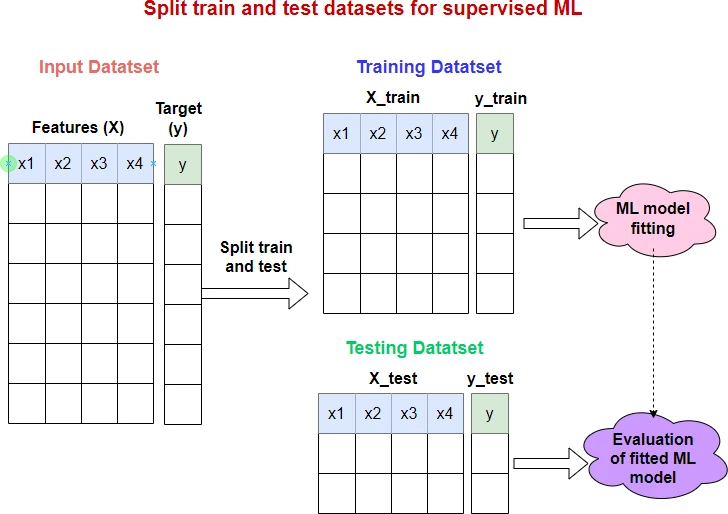# How to Split Data into Train and Test Sets in Python with sklearn

In a machine learning (ML) framework, the train and test split of input datasets is a key method for unbiased evaluation of the predictive performance of the fitted (trained) model.

In Python, the train and test split can be performed using the `train_test_split()` function from the sklearn package.

The basic syntax of `train_test_split()` is as follows:

``````# import package
from sklearn.model_selection import train_test_split

X_train, X_test, y_train, y_test = train_test_split(*arrays)
``````

By default, `train_test_split()` splits the data as 75% for the training set and 25% for the test set.

In addition to arrays, `train_test_split()` also accepts pandas Dataframe or scipy-sparse matrices as input.The following four examples will help you understand how to use `train_test_split()` function for splitting train and test datasets,

## Example 1

Generate random datasets as arrays with `X` features and `y` target variable, and split them with a default proportion of 75% train and 25% test datasets.

By default, the input datasets will be shuffled (random sampling without replacement) before splitting.

``````import numpy as np
from sklearn.model_selection import train_test_split

# create X and y arrays
X = np.random.randint(100, size=20).reshape((10, 2))
# output
array([[94, 88],
[21, 78],
[ 2, 70],
[42, 60],
[42,  7],
[16, 83],
[85, 78],
[38, 46],
[95, 61],
[95, 88]])

y = np.random.randint(100, size=10)
# output
array([ 8, 34,  9, 63, 42, 43, 31, 65, 53, 43])

# split train and test
# Set random_state to any int for reproducible output
X_train, X_test, y_train, y_test = train_test_split(X, y, random_state=0)
X_train
# output
array([[95, 61],
[94, 88],
[ 2, 70],
[95, 88],
[38, 46],
[85, 78],
[42,  7]])

y_train
# output
array([53,  8,  9, 43, 65, 31, 42])

X_test
# output
array([[16, 83],
[21, 78],
[42, 60]])

y_test
# output
array([43, 34, 63])
``````

In the above example, the `X` and `y` datasets were split into 75% train and 25% test datasets.

## Example 2

In this example, we will change the test proportion to 20% (set `test_size`=0.2). The `train_size` parameter will be automatically set to complement of `test_size` i.e. here `train_size`=0.8

In addition, we will not perform the shuffling of the dataset before splitting (set `shuffle`=False).

``````import numpy as np
from sklearn.model_selection import train_test_split

# create X and y arrays
X = np.random.randint(100, size=20).reshape((10, 2))
# output
array([[25, 71],
[91, 83],
[18, 33],
[31, 98],
[89, 34],
[13, 81],
[35, 92],
[46, 56],
[59, 85],
[43, 18]])

y = np.random.randint(100, size=10)
# output
array([64, 92, 59, 85,  0, 29, 29, 35, 88, 56])

# split train and test
X_train, X_test, y_train, y_test = train_test_split(X, y, test_size=0.2,
shuffle=False, random_state=0)
X_train
# output
array([[25, 71],
[91, 83],
[18, 33],
[31, 98],
[89, 34],
[13, 81],
[35, 92],
[46, 56]])

y_train
# output
array([64, 92, 59, 85,  0, 29, 29, 35])

X_test
# output
array([[59, 85],
[43, 18]])

y_test
# output
array([88, 56])
``````

## Example 3

In some ML datasets, the target variable is highly imbalanced. For example, in binary classification, the positive classes could be extremely high in number than the negative classes or vice versa. In such cases, stratified sampling should be used while splitting the datasets.

The stratified sampling will ensure that the training and testing datasets will have a similar proportion of the target classes as in the original input dataset.

To enable stratified split in `train_test_split()`, you should use `stratify` parameter. When the `stratify` parameter is used, the `shuffle` parameter must be `True`.

``````import numpy as np
from sklearn.model_selection import train_test_split

# create X and y arrays
X = np.random.randint(100, size=20).reshape((10, 2))
# output
array([[64,  8],
[ 3, 98],
[26, 71],
[55, 36],
[32, 71],
[62, 99],
[82, 60],
[74,  3],
[50, 35],
[71, 80]])

y = np.random.randint(2, size=10)
# output
array([1, 1, 0, 1, 0, 0, 1, 0, 0, 0])

# split train and test
X_train, X_test, y_train, y_test = train_test_split(X, y, stratify=y, random_state=0)
X_train
# output
array([[74,  3],
[62, 99],
[32, 71],
[82, 60],
[64,  8],
[71, 80],
[55, 36]])

y_train
# output
array([0, 0, 0, 1, 1, 0, 1])

X_test
# output
array([[26, 71],
[50, 35],
[ 3, 98]])

y_test
# output
array([0, 0, 1])
``````

## Example 4

In this example, we will use pandas DataFrame as input and split them in proportion of 75% train and 25% test datasets (default split ratio).

``````import pandas as pd
from sklearn.model_selection import train_test_split

# output
age    BMI  glucose  diagn
0   48  23.50       70      1
1   45  26.50       92      2
2   86  27.18      138      2
3   86  21.11       92      1
4   68  21.36       77      1

# split train and test
df_train, df_test = train_test_split(df, random_state=0)

df_train
# output
age    BMI  glucose  diagn
3   86  21.11       92      1
2   86  27.18      138      2
4   68  21.36       77      1

df_test
# output
age   BMI  glucose  diagn
0   48  23.5       70      1
1   45  26.5       92      2
``````

## Subscribe to get new article to your email when published

* indicates required